# Consider the isothermal compression of 1 mole of a monatomic ideal gas, initially at a pressure...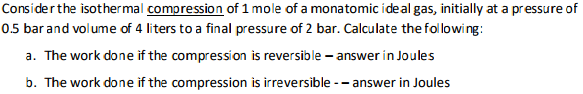Consider the isothermal compression of 1 mole of a monatomic ideal gas, initially at a pressure of 0.5 bar and volume of 4 liters to a final pressure of 2 bar. Calculate the following: a. The work done if the compression is reversible-answer in Joules b. The work done if the compression is irreversible-answer in Joules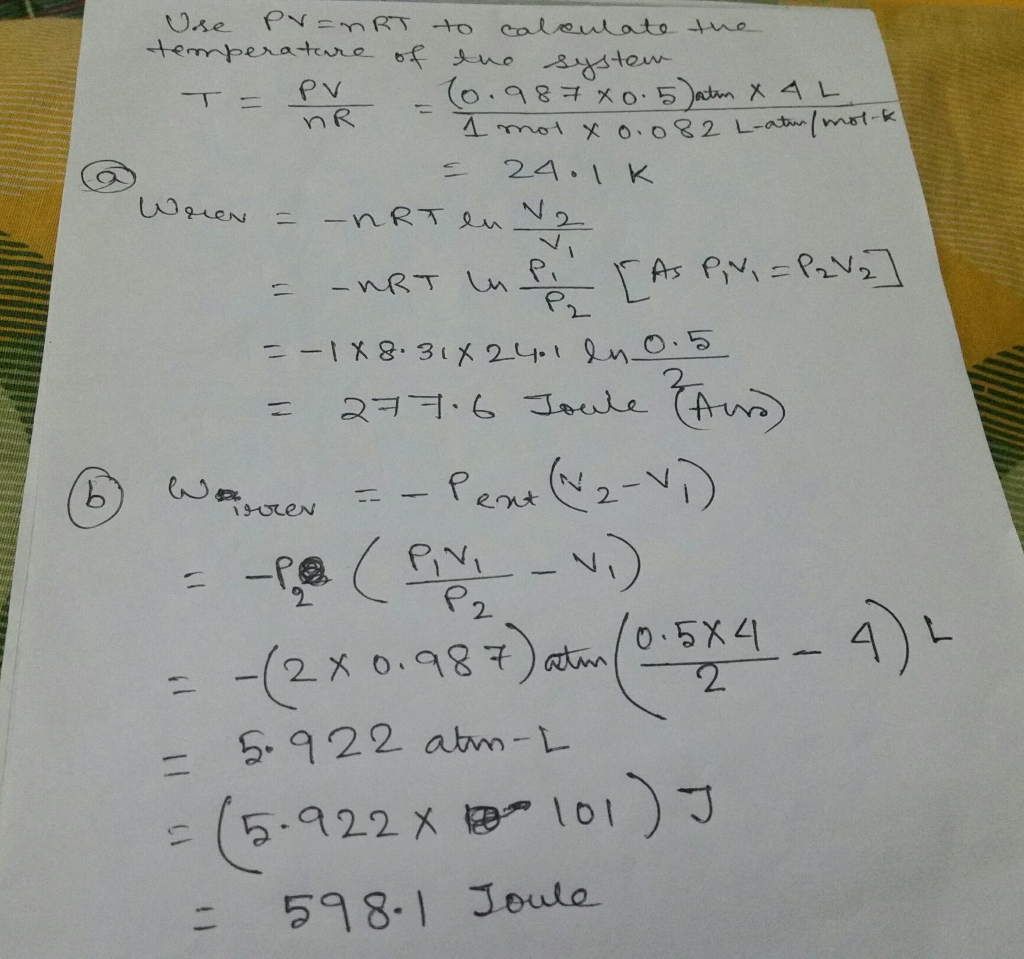#### Earn Coin

Coins can be redeemed for fabulous gifts.

Similar Homework Help Questions
• ### One mole of an ideal monatomic gas initially at 300 K is expanded from an initial pressure of 10 atm to a final pressure of 1 atm

One mole of an ideal monatomic gas initially at 300 K is expanded from an initial pressure of10 atm to a final pressure of 1 atm. Calculate ��E, q, w, ��H, and the final temperature T2 forthis expansion carried out according to each of the following paths. The molar heat capacityat constant volume for a monatomic gas is CV = 3/2 nR. Taken from Tinoco 2.8.(a) An isothermal, reversible expansion.(b) An expansion against a constant external pressure of 1 atm...

• ### An ideal monatomic gas initially has a temperature of 267 K and a pressure of 6.14...

An ideal monatomic gas initially has a temperature of 267 K and a pressure of 6.14 atm. It is to expand from volume 488 cm3 to volume 1610 cm3. If the expansion is isothermal, what are (a) the final pressure and (b) the work done by the gas? If, instead, the expansion is adiabatic, what are (c) the final pressure and (d) the work done by the gas?

• ### An ideal monatomic gas initially has a temperature of T and a pressure of p. It is to expand...

An ideal monatomic gas initially has a temperature of T and a pressure of p. It is to expand from volume V1 to volume V2. If the expansion is isothermal, what are thefinal pressure pfi and the work Wi done by the gas? If, instead, the expansion is adiabatic, what are the final pressure pfa and the work Wa done by the gas? Stateyour answers in terms of the given variables.

• ### Part D please An ideal monatomic gas initially has temperature Ti and pressure pi. It is...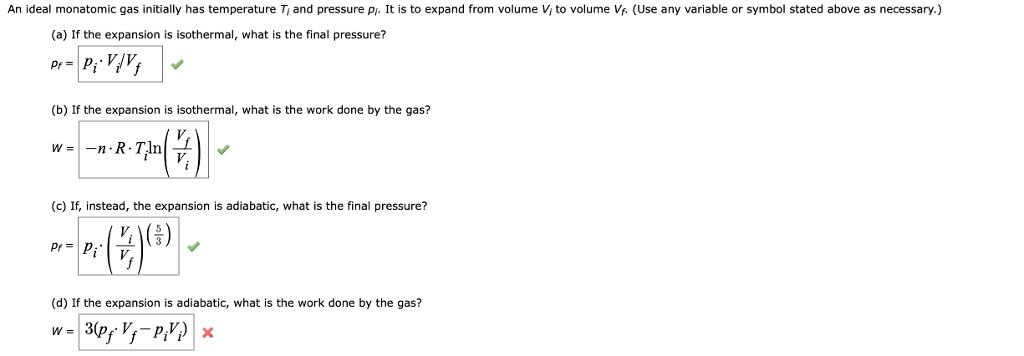Part D please An ideal monatomic gas initially has temperature Ti and pressure pi. It is to expand from volume V to volume Vf. (Use any variable or symbol stated above as necessary.) (a) If the expansion is isothermal, what is the final pressure? (b) If the expansion is isothermal, what is the work done by the gas? 42) 1219 (c) If, instead, the expansion is adiabatic, what is the final pressure? (d) If the expansion is adiabatic, what is...

• ### 6. (25 points) One mole of a monatomic ideal gas, initially at pressure P1 = 105...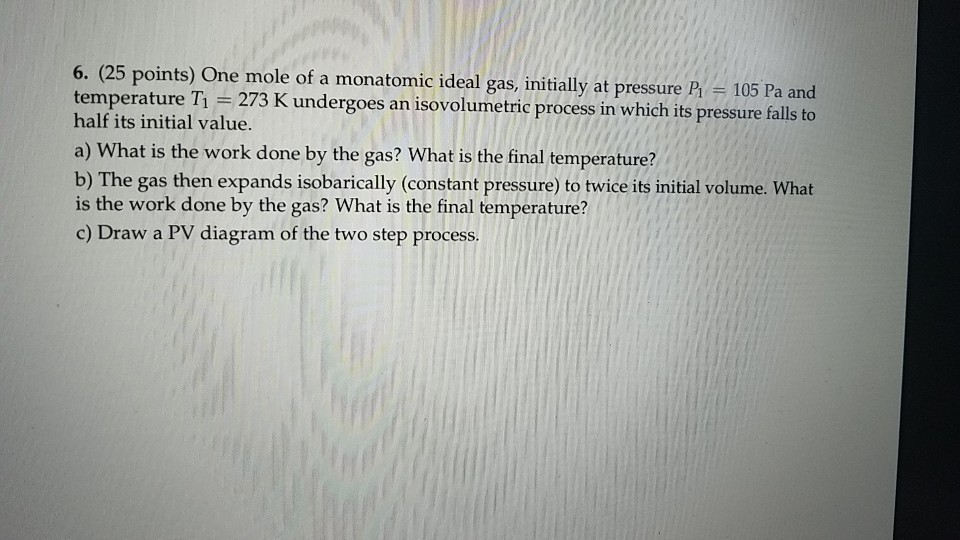6. (25 points) One mole of a monatomic ideal gas, initially at pressure P1 = 105 Pa and temperature T1 = 273 K undergoes an isovolumetric process in which its pressure falls to half its initial value. a) What is the work done by the gas? What is the final temperature? b) The gas then expands isobarically (constant pressure) to twice its initial volume. What is the work done by the gas? What is the final temperature? c) Draw a...

• ### A monatomic ideal gas that is initially at a pressure of 1.54 times 10^5 Pa and...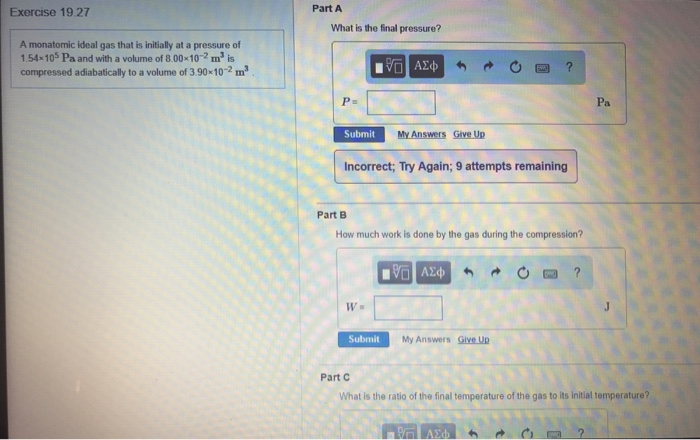A monatomic ideal gas that is initially at a pressure of 1.54 times 10^5 Pa and with a volume of 8.00 times 10^-2 m^3 is compressed adiabatically to a volume of 3.90 times 10^-2 m^3. What is the final pressure? P = ______ Pa How much work is done by the gas during the compression? W = ________ J What is the ratio of the final temperature of the gas to its initial temperature?

• ### One mole of an ideal monatomic gas, initially at a pressure of 1.04 atm and a...

One mole of an ideal monatomic gas, initially at a pressure of 1.04 atm and a volume of 0.0124 m^3 , , is heated to a final state where the pressure is 3.04 atm and the volume is 0.0274 m^3 . The gas constant is 8.31447 J/mol · K . Determine the change in entropy for this

• ### A monatomic ideal gas is initially at volume, pressure, temperature (Vi, Pi, Ti). Consider two different...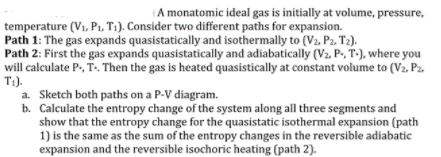A monatomic ideal gas is initially at volume, pressure, temperature (Vi, Pi, Ti). Consider two different paths for expansion. Path 1: The gas expands quasistatically and isothermally to (Va, Pz. T2) Path 2: First the gas expands quasistatically and adiabatically (V2, P.,T-),where you will calculate P T. Then the gas is heated quasistically at constant volume to (Va. P2 T1). a. Sketch both paths on a P-V diagram. b. Calculate the entropy change of the system along all three segments...

• ### 12. 1 mole of an ideal gas undergoes an isothermal expansion from V1 = 1.4L followed...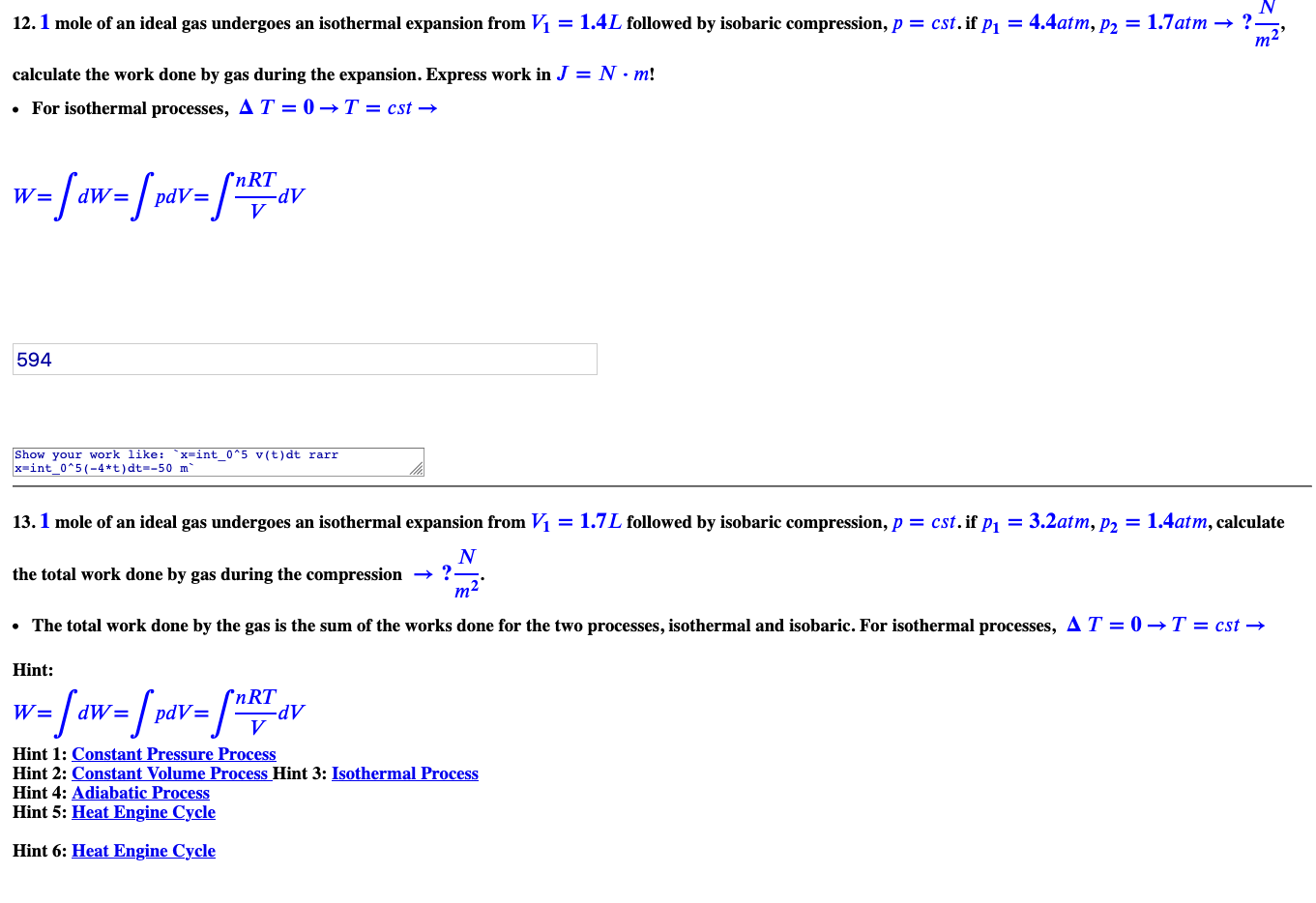12. 1 mole of an ideal gas undergoes an isothermal expansion from V1 = 1.4L followed by isobaric compression, p = cst.if P1 = 4.4atm, p2 = 1.7atm → ?- m calculate the work done by gas during the expansion. Express work in J = N·m! • For isothermal processes, AT = 0 T = cst → w=faw=fr&v=/MRT AV 594 Show your work like: `x-int_0^5 v(t)dt rarr x-int_0^5(-4*t)dt=-50 m 13. 1 mole of an ideal gas undergoes an isothermal expansion...

• ### Problem 8: Consider the reversible Carnot's cycle of an ideal monatomic gas in the cold cylinder...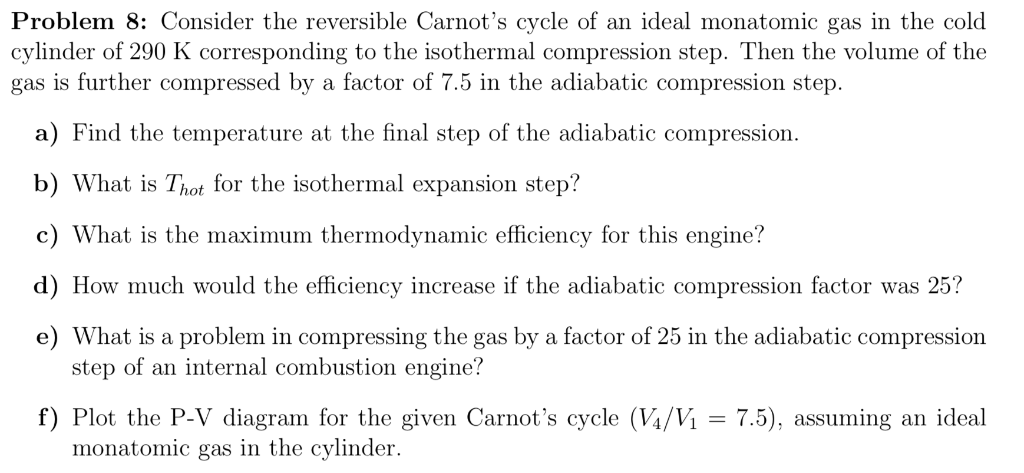Problem 8: Consider the reversible Carnot's cycle of an ideal monatomic gas in the cold cylinder of 290 K corresponding to the isothermal compression step. Then the volume of the gas is further compressed by a factor of 7.5 in the adiabatic compression step. a) Find the temperature at the final step of the adiabatic compression. b) What is Thot for the isothermal expansion step? c) What is the maximum thermodynamic efficiency for this engine? d) How much would the...

Free Homework App